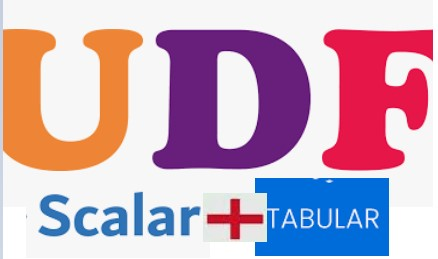During this post we will discuss about the Scalar and Tabular UDF in Snowflake. By default, a UDF is scalar, returns at most one row, consisting of a single column/value. Table UDF, Returns a set of rows with one or more columns, which can then be accessed in the FROM clause of a query. Assuming we are aware about these UDF’s, hence will discuss a USE CASE instead of going to the basics. We will see how we can use SCALAR and TABULAR UDF in SELECT clause.

Consider the scenario where we must use both type of UDF in single query statement. Later on, we will develop a view based on the output of these UDF. Also, we will see how we can use CONTAINS function in UDF to validate if INPUT value exists in Table or not.

CONTAINS: Returns a BOOLEAN. The value is True if expr2 is found inside expr1. Returns NULL if either input expression is NULL. Otherwise, returns False.

We have created below four tables, where key_info contains the KEY details. As per ask we will fetch the key id from the key_info tables and perform lookup into the respective LOOKUP tables.

#### Below diagram shows:

• Get the Orderid and extract Unitprice and Quantity from order_lkp
• Match the City and extract Customer name from cust_City_lkp
• Match the States and Codes and extract the states from state_code_lkp

Following four tables got created along with the data as below:

`create or replace table key_info(city varchar(10),states varchar(20),codes varchar(10),order_id integer);`

`create or replace table Cust_City_Lkp (Customer_name varchar(100),  city varchar(10));`

`create or replace table order_Lkp (UnitPrice integer,Quantity integer,order_id integer);`

`create or replace table state_code_lkp (Roles varchar,states varchar,codes varchar);`

Create the First Scalar UDF Function:

/* This UDF will accept CITY as input and returns CUSTOMER_NAME*/

`create or replace function Customers_By_City(ct varchar)`

`returns varchar`
``` language sql```

`as`

`\$\$`

`select any_value(Customer_name) from Cust_City_Lkp where city = ct`

`\$\$;`

Create the second Scalar UDF Function:

/* This UDF will accept ORDER_ID as input and returns UnitPrice*Quantity */

`create or replace function order_qty(id integer)`

`returns integer`

`language sql`

`as`

`\$\$`

`select any_value(UnitPrice*Quantity) from order_Lkp where order_id = id`

`\$\$;`

Create the TBULAR UDF Function:

/* This UDF will accept STATE and CODES as input and returns STATE and CODES and ROLES..

Also we are using CONTAINS function to check STATE in COLUMN

e.g. AL in (‘AL,CA,DC,DE,FL,GA,LA,MD,MS,MT’) */

`CREATE OR REPLACE FUNCTION ST_CD(ST VARCHAR,CD VARCHAR)`

`RETURNS TABLE(ST_CD_RETURN VARCHAR,states varchar,Roles varchar)`

`AS`

`\$\$`

`SELECT IFF(CONTAINS(states,ST) = TRUE,ST,`

`IFF(CONTAINS(codes,CD)=TRUE,CD,'DEFAULT VALUES')) AS ST_CD_RETURN ,states,Roles`

`FROM state_code_lkp`

`WHERE CONTAINS(CURRENT_ROLE(),Roles) = TRUE`

`\$\$`

Call the both UDF in single SELECT statement in below way and see the output:

`select a.states,a.codes,Customers_By_City(city) AS Customer_Name,order_qty(order_id),ST_CD_RETURN,b.states,b.roles from key_info a join table(ST_CD(states, CODES)) b`5 Star
100%
4 Star
0%
3 Star
0%
2 Star
0%
1 Star
0%

## 2 thoughts on “Snowflake: SCALAR and TABULAR UDF in SELECT query”

1.Ward says:

Your post SAVED ME for how to properly create a SQL scalar UDF that can pass a column to the function! I think the any_value() part in the function was the key thing to prevent the “Unsupported subquery type cannot be evaluated” error I was getting. Thanks very much!

1.Sachin Mittal says: